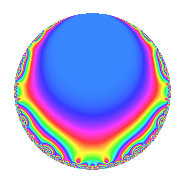# Properties

 Label 8046.2.a.cLevel 8046 Weight 2 Character orbit 8046.a Self dual Yes Analytic conductor 64.248 Analytic rank 0 Dimension 2 CM No Inner twists 1

# Related objects

## Newspace parameters

 Level: $$N$$ = $$8046 = 2 \cdot 3^{3} \cdot 149$$ Weight: $$k$$ = $$2$$ Character orbit: $$[\chi]$$ = 8046.a (trivial)

## Newform invariants

 Self dual: Yes Analytic conductor: $$64.2476334663$$ Analytic rank: $$0$$ Dimension: $$2$$ Coefficient field: $$\Q(\sqrt{3})$$ Coefficient ring: $$\Z[a_1, \ldots, a_{11}]$$ Coefficient ring index: $$1$$ Fricke sign: $$-1$$ Sato-Tate group: $\mathrm{SU}(2)$

## $q$-expansion

Coefficients of the $$q$$-expansion are expressed in terms of $$\beta = \sqrt{3}$$. We also show the integral $$q$$-expansion of the trace form.

 $$f(q)$$ $$=$$ $$q - q^{2} + q^{4} + 2 \beta q^{5} + ( 1 - 2 \beta ) q^{7} - q^{8} +O(q^{10})$$ $$q - q^{2} + q^{4} + 2 \beta q^{5} + ( 1 - 2 \beta ) q^{7} - q^{8} -2 \beta q^{10} + ( -3 + \beta ) q^{11} + ( 2 + 2 \beta ) q^{13} + ( -1 + 2 \beta ) q^{14} + q^{16} + ( 5 + \beta ) q^{17} + ( -2 - 2 \beta ) q^{19} + 2 \beta q^{20} + ( 3 - \beta ) q^{22} + ( 6 - \beta ) q^{23} + 7 q^{25} + ( -2 - 2 \beta ) q^{26} + ( 1 - 2 \beta ) q^{28} -\beta q^{29} + q^{31} - q^{32} + ( -5 - \beta ) q^{34} + ( -12 + 2 \beta ) q^{35} + ( 1 + \beta ) q^{37} + ( 2 + 2 \beta ) q^{38} -2 \beta q^{40} + ( -4 + 3 \beta ) q^{41} + ( 2 + 4 \beta ) q^{43} + ( -3 + \beta ) q^{44} + ( -6 + \beta ) q^{46} + ( 4 + 2 \beta ) q^{47} + ( 6 - 4 \beta ) q^{49} -7 q^{50} + ( 2 + 2 \beta ) q^{52} + ( 8 - \beta ) q^{53} + ( 6 - 6 \beta ) q^{55} + ( -1 + 2 \beta ) q^{56} + \beta q^{58} + ( -5 + \beta ) q^{59} + ( 1 + \beta ) q^{61} - q^{62} + q^{64} + ( 12 + 4 \beta ) q^{65} + ( -1 - 3 \beta ) q^{67} + ( 5 + \beta ) q^{68} + ( 12 - 2 \beta ) q^{70} + ( -2 - 7 \beta ) q^{71} + ( -2 + 2 \beta ) q^{73} + ( -1 - \beta ) q^{74} + ( -2 - 2 \beta ) q^{76} + ( -9 + 7 \beta ) q^{77} + ( -11 - \beta ) q^{79} + 2 \beta q^{80} + ( 4 - 3 \beta ) q^{82} + ( -7 + 3 \beta ) q^{83} + ( 6 + 10 \beta ) q^{85} + ( -2 - 4 \beta ) q^{86} + ( 3 - \beta ) q^{88} + ( -6 + \beta ) q^{89} + ( -10 - 2 \beta ) q^{91} + ( 6 - \beta ) q^{92} + ( -4 - 2 \beta ) q^{94} + ( -12 - 4 \beta ) q^{95} + ( 9 + \beta ) q^{97} + ( -6 + 4 \beta ) q^{98} +O(q^{100})$$ $$\operatorname{Tr}(f)(q)$$ $$=$$ $$2q - 2q^{2} + 2q^{4} + 2q^{7} - 2q^{8} + O(q^{10})$$ $$2q - 2q^{2} + 2q^{4} + 2q^{7} - 2q^{8} - 6q^{11} + 4q^{13} - 2q^{14} + 2q^{16} + 10q^{17} - 4q^{19} + 6q^{22} + 12q^{23} + 14q^{25} - 4q^{26} + 2q^{28} + 2q^{31} - 2q^{32} - 10q^{34} - 24q^{35} + 2q^{37} + 4q^{38} - 8q^{41} + 4q^{43} - 6q^{44} - 12q^{46} + 8q^{47} + 12q^{49} - 14q^{50} + 4q^{52} + 16q^{53} + 12q^{55} - 2q^{56} - 10q^{59} + 2q^{61} - 2q^{62} + 2q^{64} + 24q^{65} - 2q^{67} + 10q^{68} + 24q^{70} - 4q^{71} - 4q^{73} - 2q^{74} - 4q^{76} - 18q^{77} - 22q^{79} + 8q^{82} - 14q^{83} + 12q^{85} - 4q^{86} + 6q^{88} - 12q^{89} - 20q^{91} + 12q^{92} - 8q^{94} - 24q^{95} + 18q^{97} - 12q^{98} + O(q^{100})$$

## Embeddings

For each embedding $$\iota_m$$ of the coefficient field, the values $$\iota_m(a_n)$$ are shown below.

For more information on an embedded modular form you can click on its label.

Label $$\iota_m(\nu)$$ $$a_{2}$$ $$a_{3}$$ $$a_{4}$$ $$a_{5}$$ $$a_{6}$$ $$a_{7}$$ $$a_{8}$$ $$a_{9}$$ $$a_{10}$$
1.1
 −1.73205 1.73205
−1.00000 0 1.00000 −3.46410 0 4.46410 −1.00000 0 3.46410
1.2 −1.00000 0 1.00000 3.46410 0 −2.46410 −1.00000 0 −3.46410
 $$n$$: e.g. 2-40 or 990-1000 Significant digits: Format: Complex embeddings Normalized embeddings Satake parameters Satake angles

## Inner twists

This newform does not admit any (nontrivial) inner twists.

## Atkin-Lehner signs

$$p$$ Sign
$$2$$ $$1$$
$$3$$ $$-1$$
$$149$$ $$1$$

## Hecke kernels

This newform can be constructed as the intersection of the kernels of the following linear operators acting on $$S_{2}^{\mathrm{new}}(\Gamma_0(8046))$$:

 $$T_{5}^{2} - 12$$ $$T_{11}^{2} + 6 T_{11} + 6$$